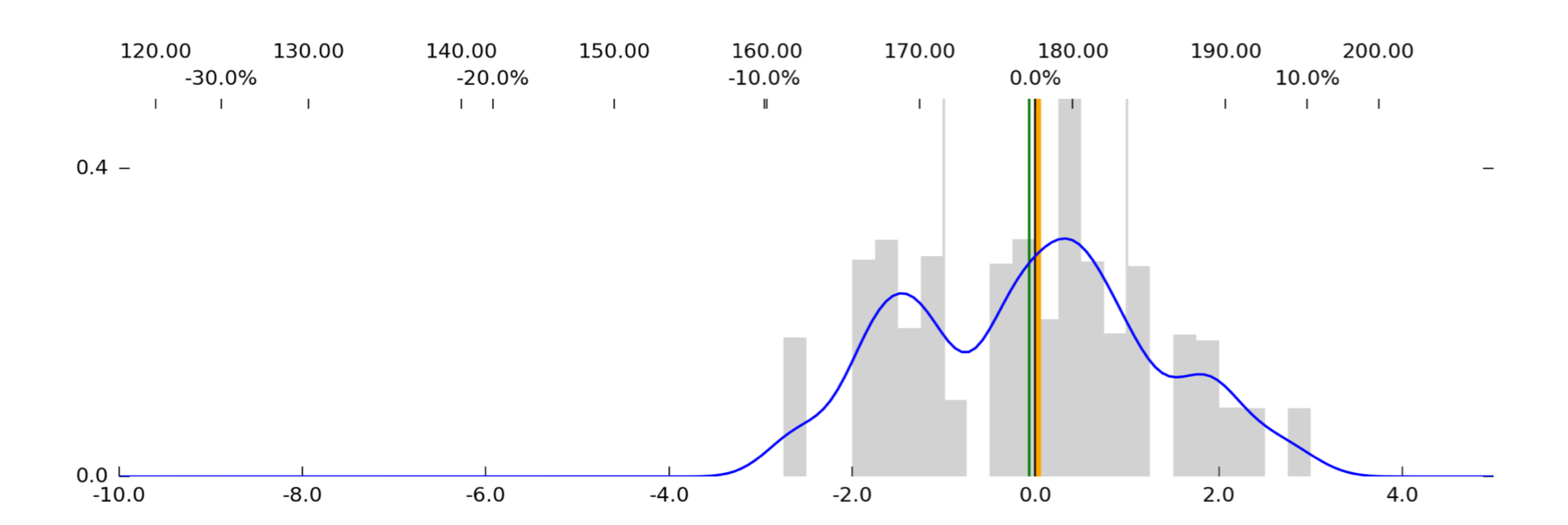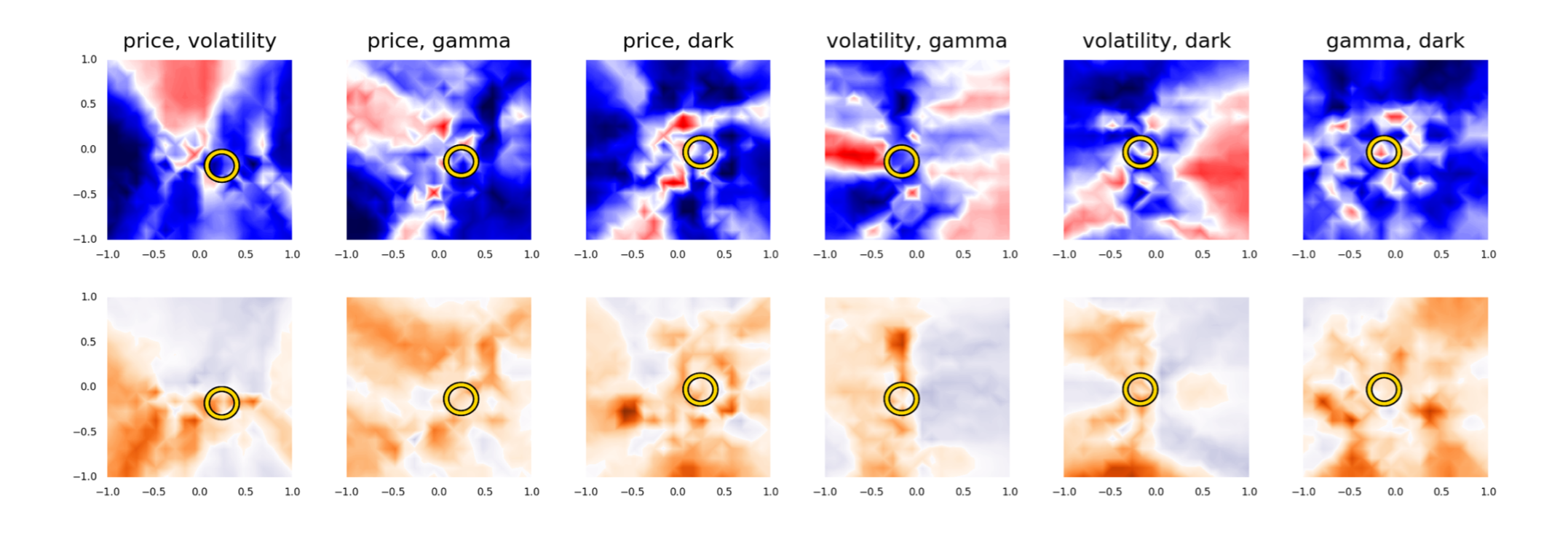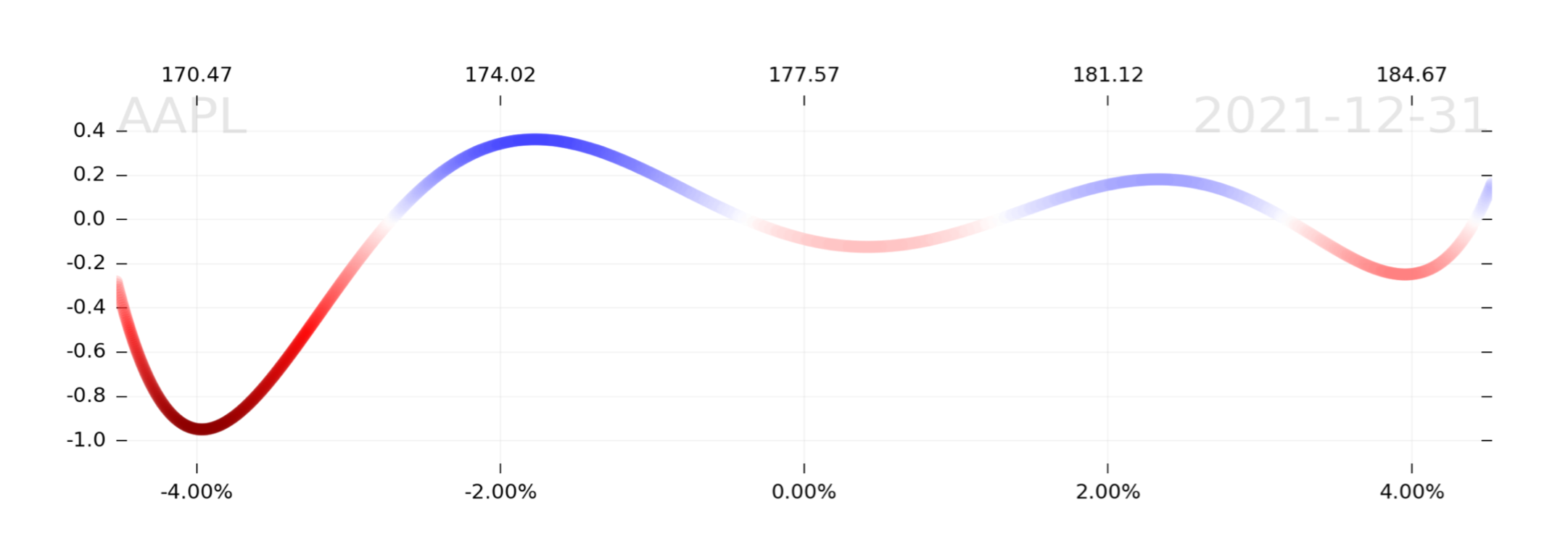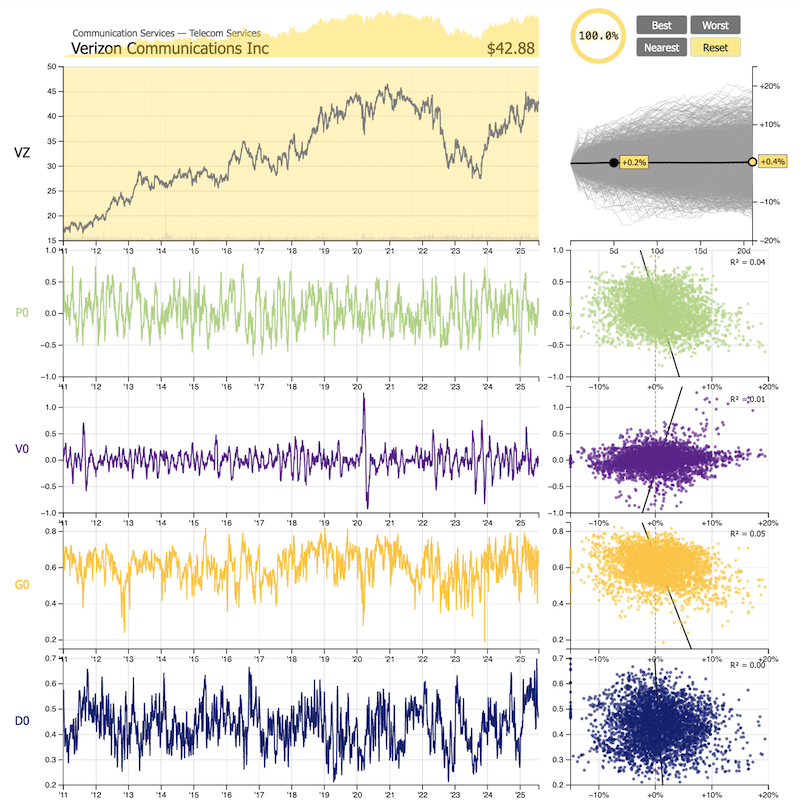## I. The Four Axes

There are four predictors that form the basis of all of our data—(a) price, (b) volatility, (c) gamma, and (d) dark—let's address each of them in turn.

#### a. Price (P)

Movement in price is usually denominated in dollars, points, or percent. Movement in price is rarely, if ever, denominated in what really matters—the price change in relation to historical volatility, i.e., the price change relative to how much it usually moves.

If two stocks, A and B, both move 5.00% tomorrow, that's not very useful information on its own. If we know, however, that Stock A has been moving 1.00% per day, on average; and Stock B has been moving 10.00% per day, on average; then we're able to place those percentage moves in context:

• Stock A moved 5.00x its average expected move.
• Stock B moved 0.50x its average expected move.

Another way to express the multiple of the expected move is in "mean absolute deviation" (MAD). A 1.00x move is a 1 MAD move. A 5.00x move is a 5 MAD move. A 5 MAD move is big.

But what if we want to get a contextual sense of how a stock has moved over not the past day, but the past month? Well, usually this, too, is denominated in percent moves. So, e.g., if a stock (priced at \$100) that usually moves 1.00% per day starts off a month with a nasty one-day, 20% decline (\$100 → \$80), then gradually claws back 1.00% per day for the next 20 market days (→ \$97.61), the "monthly return" is -2.38%. But that's... not very useful information, because it doesn't at all describe the magnitude of the tail events we experienced during the month.

Rather than refer to this monthly return, we want to have some metric that considers each of the daily moves that comprise the past month. But, like we said before, daily percent moves just aren't able to provide enough context on their own. In order to get the context we need, we have to compare every day in the past month to the trailing historical daily moves at the time. So if the 20% decline followed a period of 1.00% average returns, then that -20% move was a 20-MAD event (!). And since the 20% decline would substantially raise the trailing historical average daily move of the past month (1.00% → 1.90%), then the next few days of 1.00% returns would be a mere 0.53 MAD.

In this context, the mean return over the period, despite 20 straight days of +0.53 MAD returns, would be a lousy -0.45 MAD. This result emphasizes the outsized impact of that 20-MAD event at the beginning of the month.

As juxtaposition, imagine that the 20% decline followed a period of 5.00% average daily moves instead. The decline would be a 4 MAD event instead (not nearly as big a deal). In this case, the subsequent mean return over the period would be not -0.45 MAD, but +0.26 MAD. Comparatively bullish!

This is why, if we only looked at percentages, we'd get the totally wrong idea. Changes in price must be viewed in the context of volatility, or else they lose meaning. And while we may know this intuitively, and we may have a sense for the way volatility impacts true returns, we have to declare all of this explicitly when we're attempting to use numerical methods. If we don't, it's garbage-in, garbage-out.

Summary: 'Price' is the 1-month sum of daily MAD returns, with a rolling window of 1-month volatility (defined as average daily moves) as its denominator. It will generally move between -1 and +1. This number tells us, better than anything else, how price has really been moving, and is comparable across any and all assets.

#### b. Volatility

Why is volatility important? Because if a stock is becoming more or less volatile, that gives us crucial context about how market participants are engaging with the stock. If volatility is decreasing while price rises, that's a very different situation from volatility increasing as price rises, and you would never want to mistake one for the other.

So, in the same way that 'price' records the change in price as a function of volatility, 'volatility' records the change in volatility as a function of volatility. Because in the same way that change in price, denominated in percent, fails to capture the reality of the change; change in volatility denominated in vol points (like VIX), also misses the mark.

Return to the example above: The average daily move of a stock, before that 20% decline, was always 1.00%. I.e., the mean absolute deviation (MAD) was 1.00%. But as we said, the subsequent 20-MAD event raised the average daily move over the past month to 1.90%. Since, a month prior, MAD was 1.00%, and suddenly, MAD became 1.90%, all we really need to do to describe the change in volatility is to difference these (1.90 - 1.00). I.e., 'volatility' is +0.90 MAD.

Like 'price', 'volatility' conveniently tends to move within the domain [-1, +1]. So a 'volatility' of +0.90 MAD means there was a huge relative increase in volatility, which is the perspective we need to go alongside 'price'.

Now we have two predictors, 'price' and 'volatility', that both use the same units (MAD), are measuring nothing more than simple averages on a 1-month period, move in the domain [-1, 1], and can be used on any asset.

This is quite powerful already, but wait—there's more!

#### c. Gamma

Ever since our 2016 paper on gamma exposure (GEX), people have been eager to replicate and extend the concept. Here, though, we're peeling away any and every layer of complexity to the computation and revealing a simple ratio: The gamma of all call open interest to the gamma of all put open interest. A call-to-put ratio, but measured in gamma.

So, e.g., if the gamma of all call open interest in a stock, across all expirations and strikes, adds up to 500,000 shares per 1.00% move; and the gamma of all of the same puts adds up to 1,000,000 shares per 1.00% move, then 'gamma' is 0.50. I.e., "there is half as much call gamma as put gamma." Likewise, if call gamma is 20,000 and put gamma is 10,000, 'gamma' is 2.00.

Any time 'gamma' is under 1.00, puts are relatively more important; any time 'gamma' is over 1.00, calls are relatively more important. By design, the critically important concept of "zero GEX" is completely preserved here (as gamma = 1.00), while removing all other confounding factors.

Why the simplification? Because we have the luxury, now, of viewing gamma in the context of both (a) 'price' and (b) 'volatility'. This gives us a much clearer view of changes in option exposures, and isolates the impact that options have in individual stocks in a way that could never be done with one-dimensional data.

And the same can be said of our fourth and final predictor:

#### d. Dark

Dark pool short volume, as reported by FINRA in its Reg SHO daily files, is the basis for the Dark Index (DIX), and the subject of the paper, "Short is Long." Like gamma, dark is a simple ratio: Short off-exchange volume is the numerator, and all off-exchange volume is the denominator.

The result can be expressed as a decimal (0.0 to 1.0) or as a percentage (like DIX), but in either case, it tells us the relative amount of trade volume marked "short" in off-exchange (dark) trading.

As with gamma, dark becomes more powerful when viewed in the context of the other predictors. Because it rises and falls without regard to price, volatility, or gamma, it provides an uncorrelated signal that frequently tracks sentiment, whether or not that sentiment has been reflected in price.

This fourth axis is the final dimension of the data. The need for each of these four dimensions as inputs is what drives the presentation and visualization of the data (it's not easy to think in 4-D), as well as the algorithmic methods with which we derive probability distributions.

Since the daily PDF documents do the most the express that multidimensionality, let's talk about those next.

## II. The PDFs

At the top-right of every Chart page, and to the right on both the Dashboard and Research page, there is a download link to the ticker's PDF summary. This includes (a) the distribution, (b) the weather maps, and (c) the step-forward analysis.

#### a. The Distribution

Feeble-minded humans can't see in four dimensions, but 100 lines of code named Robot Jim can.

Consider each of our four predictors as an axis on a 4-D scatter plot, "color" every historical data point according to its 1-week return, and then search for the historical combination of data coordinates that most closely resembles the current scenario.

For example, if price is currently up, volatility is currently down, gamma is somewhere in the middle, and dark is extremely high; then we want to look for every historical analog, where price was up, vol was down, gamma was middling, and dark was high. Then (as if four dimensions of data plus returns wasn't enough), we want to weight the historical returns data to emphasize data that is both nearer in time, and nearer in space, to the current coordinates.

This weighted distribution, containing 42 discrete historical events (two months of market-day data are being sampled here), their weights, and their 1-week return, is then plotted as a histogram, smoothed with kernel density estimation, and consulted for its mean and median expected returns—which are plotted on the x-axis in green and orange, respectively.As with the 'price' data, the x-axis is standardized to MAD returns, so a +1.00 MAD gain would be a positive return that matches the average expected weekly move in the stock, and a -2.00 MAD return would be a weekly loss twice the expected move. For context, this is converted into both percent and spot prices on the upper x-axis.

By default, the range on the x-axis is -10 to +5 MAD for consistency (and because most stocks have left-skewed returns), but the axis is extended whenever higher-magnitude returns need to be shown.

#### b. The Weather Maps

We can't do what Robot Jim does, because we can only see in three dimensions, but we can come pretty close to what he does, by taking multiple cross-sections of pair-wise predictors and their historical returns data. Since there are four data axes, there are six possible pairs of predictors, and each of these predictors is plotted, on a fully normalized basis [-1, 1]. with each of the others.This way, we should be able to immediately get a sense of whether there are historical patterns and where they occur. Are there any particularly bold, or extensive, red or blue blotches on the map? These tell us that that particular combination of predictors has a notable historical pattern. Perhaps 1-week returns are bullish when dark is high and gamma is low—or maybe it's the opposite!

But we really need to know about more than direction—magnitude matters just as much. And so, a second set of weather maps tells us whether 1-week returns tend to land under, or over, the 1.00 MAD expected move. I.e., does volatility tend to increase (orange) or decrease (purple)?

This means that any given coordinate pair can be associated with price-up, vol-up; price-up, vol-down; price-down, vol-up; or price-down, vol-down. By way of example, see how the upper-right corner of AAPL's gamma, dark weather map not only corresponds to strongly positive returns (blue), but also an increase in volatility (orange)! Probably because high gamma means a lot of calls have been bought, and high dark means customers are buying tons of shares in off-exchange transactions. Yay, bullish!

#### c. The Step-forward

This is the part where we have to do a bit of time-traveling. See, of all of our predictors, one of them, dark, really can't be known until after the market closes, because FINRA's data doesn't update until around 5:30pm. This means that we can't even take an educated guess at what dark will be until after 4pm.

And so, in order to make it possible to backtest our data in the most conservative way possible (such that you are never accidentally including that 1.5 hours of lookahead bias), we lag the dark data by a full day. This means that, for any day in history, our signal can be assumed to be able to be traded during the day, or at the close, because we're not depending on that 5:30pm data.

The only reason this comes up, of course, is that we believe we can derive a number for each of the other three predictors during the day, and before the market close. It just takes a bit of... counterfactual modeling.

Specifically, what needs to be done is to compute, for all possible future closing prices of a stock, the future price, volatility, and gamma values. In the case of price and volatility, this is a matter of imagining how the paths of price and volatility returns would change given a range of closing prices (pretty easy); but in the case of gamma, it's a matter of computing thousands of simulated changes in price and implied volatility for every option with any open interest, updating open interest as new data becomes available, and then decaying every option's time value by a day while you're at it (not so easy).

But when you combine all this counterfactual modeling, you end up with a fairly high-confidence method for determining exactly what closing prices would have what kind of impact on subsequent forecasts. I.e., we should be able to know the day's closing forecast (future expected mean) before the 4pm close.The step-forward curve tells us exactly this: The wiggly blue-and-red curve is the mean 1-week forward return that we'd expect following the potential next-day returns on the x-axis. In the example above, the most bullish outcome for the stock would be a 2.00% next-day loss, which would predict subsequent strength (+0.4 MAD). The most bearish outcome would be a 4.00% next-day loss, which would predict further losses (-1.0 MAD).

For all this talk of time-traveling, though, the PDF document is really nothing more than a point-in-time analysis of the day's data. To really get a feel for the history of a stock, and how it relates to the data, we need some good old-fashioned time-series charts.

## III. The ChartsThese are fast, interactive charts, designed to cram over ten years and 15 time-series onto just one x-axis and two y-axes. Functionality is as minimal as possible.

• click-and-drag to zoom in on an area
• double-click to zoom back out
• mouse over to see more detailed data
• click on the key to the right to hide and show

And that's all there is to it. Fast charts, no frills. The data on the charts is described below, with their spreadsheet column names in parentheses.

1. Open, High, Low, Close (OPEN, HIGH, LOW, CLOSE):
Pretty self-explanatory, right? We like candlesticks. You should too.
2. Price (P):
The price predictor, as described above, normalized to the [-1, 1] domain.
3. Volatility (V):
The volatility predictor, as described above, normalized to the [-1, 1] domain.
4. Gamma (G):
The gamma predictor, as described above, normalized to the [-1, 1] domain.
5. Dark (D):
The dark predictor, as described above, normalized to the [-1, 1] domain.
(The non-normalized predictors are in spreadsheet columns P0, V0, G0, and D0.)
6. Mean (MEAN_SPOT):
The average price of Robot Jim's 1-week forecast.
7. xx Mean (xx_MEAN_SPOT):
The average price of Robot Jim's 1-week forecast for the denoted pair. E.g., "PV Mean" is the forecast of only the price, volatility pair.

The charts are meant to provide just enough historical context to understand what the patterns visible in the weather maps really mean, and how events actually unfold. But some of you will want to test your own signals, create your own moving averages, look for your own patterns, and run your own backtests. And that brings us to...

## IV. The Sheets (and the API)

Every security in our database can be exported as a spreadsheet document (CSV). Here are the columns in those spreadsheets, and some numerical methods for extending the data:

• DATE:
YYYY-MM-DD
• TIMESTAMP:
Unix time, seconds from epoch.
• MEAN:
Robot Jim's mean 1-week forecast, expressed in mean absolute deviation (MAD).
• MEAN_SPOT:
Robot Jim's mean 1-week forecast, expressed in stock price.
• MEDIAN:
Robot Jim's median 1-week forecast, expressed in MAD.
• VOL:
Robot Jim's mean 1-week volatility forecast, expressed in MAD.
• VOL_MEDIAN:
Robot Jim's median 1-week volatility forecast, expressed in MAD.
• MEAN_PCT:
Robot Jim's mean 1-week forecast, expressed in percent.
• MEDIAN_PCT:
Robot Jim's median 1-week forecast, expressed in percent.
• VOL_PCT:
Robot Jim's mean 1-week volatility forecast, expressed in percent.
• VOL_MEDIAN_PCT:
Robot Jim's median 1-week volatility forecast, expressed in percent.
• P0:
The 'price' predictor, not normalized.
• V0:
The 'volatility' predictor, not normalized.
• G0:
The 'gamma' predictor, not normalized.
• D0:
The 'dark' predictor, not normalized.
• P:
The 'price' predictor, normalized to [-1, 1].
• V:
The 'volatility' predictor, normalized to [-1, 1].
• G:
The 'gamma' predictor, normalized to [-1, 1].
• D:
The 'dark' predictor, normalized to [-1, 1].
• xx_MEAN:
Robot Jim's mean 1-week forecast derived exclusively from the 'xx' pair, expressed in mean absolute deviation (MAD).
• xx_MEAN_SPOT:
Robot Jim's mean 1-week forecast derived exclusively from the 'xx' pair, expressed in stock price.
• xx_MEDIAN:
Robot Jim's median 1-week forecast derived exclusively from the 'xx' pair, expressed in MAD.
• xx_VOL:
Robot Jim's mean 1-week volatility forecast derived exclusively from the 'xx' pair, expressed in MAD.
• xx_VOL_MEDIAN:
Robot Jim's median 1-week forecast derived exclusively from the 'xx' pair, expressed in MAD.
This is the 1-month trailing realized volatility (daily), expressed in mean absolute deviation (MAD). As such, it is also the conversion factor for turning a MAD-denominated number into a percent. E.g., if you'd like to have a "PV_MEDIAN_PCT" column (which is not in this data), simply multiply "PV_MEDIAN" by "1MAD_PCT".
The conversion factor for turning a MAD-denominated number into a spot price. E.g., if you'd like to have a "PV_MEDIAN_SPOT" column (which is not in this data), simply multiply "PV_MEDIAN" by "1MAD_SPOT".
• IV:
A rough 30-day implied volatility, expressed an an annualized standard deviation (standard).
• IV_PCT:
A rough 30-day implied volatility, expressed as a 1-week average expected move (%).
• IV_USD:
A rough 30-day implied volatility, expressed as a 1-week average expected move (\$).
• OPEN, HIGH, LOW, CLOSE:
Nothing fancy.
• VOLUME:
Total (lit and dark, all tapes) daily volume.

This data is meant to be extensive and extensible. With the two conversion factors ("1MAD_x") and implied volatility (IV), every mean return, median return, and volatility can be placed in the context of historical and implied vol. With the availability of mean, median, and vol returns, split by predictor pair, the efficacy of innumerable mix-and-match strategies can be evaluated. Does AAPL perform better with a strategy that focuses on the price, volatility relationship, or on the gamma, dark relationship? Et cetera.

At this point, we ought to mention the API, because if you plan on doing any such extensive testing, you'll want batch processing and programmatic access. More on that here.

Now, finally, let's talk about the tiny bit of data that gets squeezed out the other end of all this insanity.

## V. The Research Page

This humble page is for context and idea generation. It searches through the top 1000 securities by dollar volume and finds the best bullish and bearish opportunities (50 from each category), then sorts them according to Robot Jim's return forecast (MEAN).

If you have the need to add some complexity to this process, or to apply your own sorting and weighting schemes, there's an API call (/latest) that returns all of the most recent day's data, in JSON or CSV format, for your nerdy pleasure.